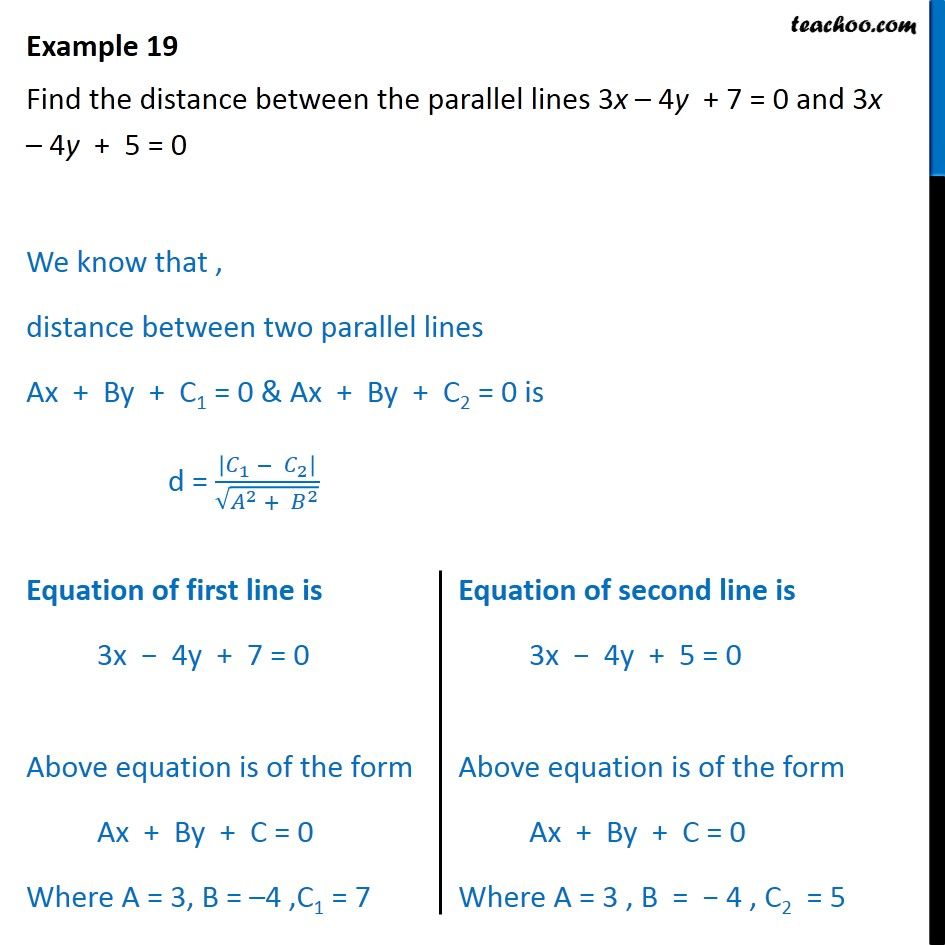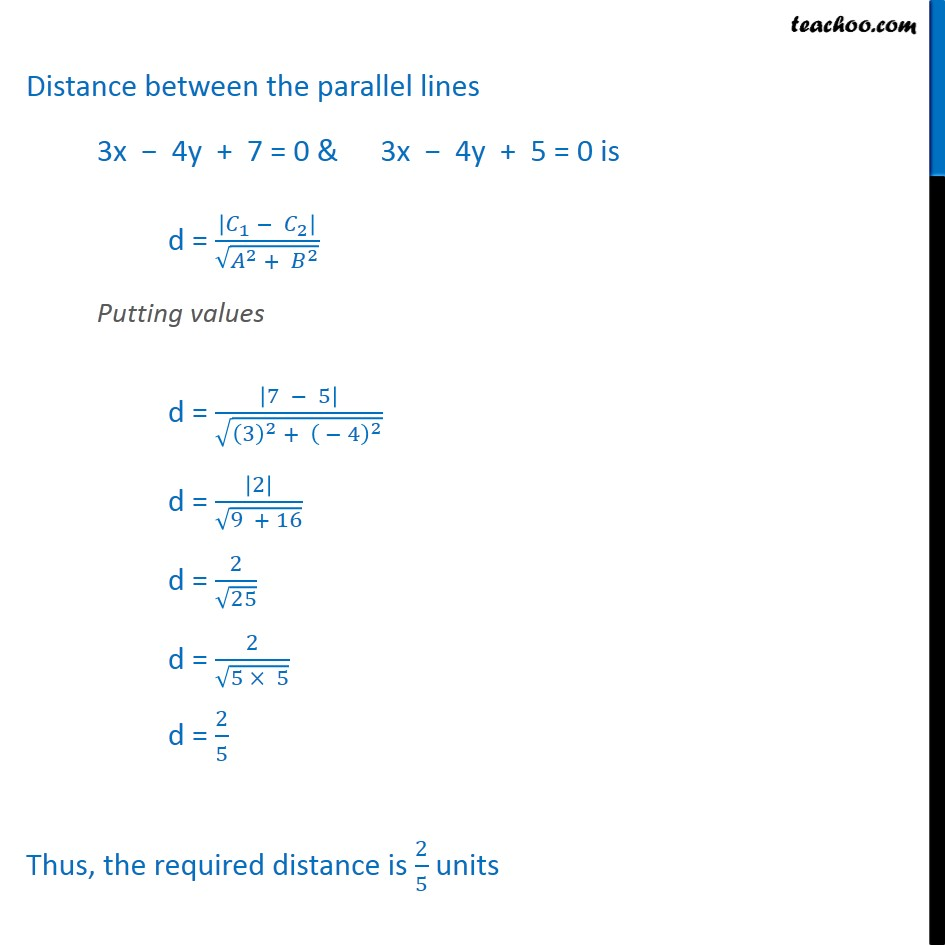Subscribe to our Youtube Channel - https://you.tube/teachoo

1. Chapter 10 Class 11 Straight Lines
2. Concept wise
3. Distance - Between two parallel lines

Transcript

Example 19 Find the distance between the parallel lines 3x 4y + 7 = 0 and 3x 4y + 5 = 0 We know that , distance between two parallel lines Ax + By + C1 = 0 & Ax + By + C2 = 0 is d = | _1 _2 |/ ( ^2 + ^2 ) Distance between the parallel lines 3x 4y + 7 = 0 & 3x 4y + 5 = 0 is d = | _1 _2 |/ ( ^2 + ^2 ) Putting values d = |7 5|/ ((3)^2 + ( 4)^2 ) d = |2|/ (9 + 16) d = 2/ 25 d = 2/ (5 5) d = 2/5 Thus, the required distance is 2/5 units

Distance - Between two parallel lines